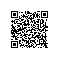# 剑指offer系列之八：跳台阶问题

package com.rhwayfun.offer;

public class JumpStep {

public int jumpFloor(int target) {
int[] r = { 0, 1, 2 };
if (target <= 2)
return r[target];

int one = 1;
int two = 2;
int result = 0;
for (int i = 3; i <= target; i++) {
result = one + two;
one = two;
two = result;
}

return result;
}

public static void main(String[] args) {
int result = new JumpStep().JumpFloorII(3);
System.out.println(result);
}
}

……

public int JumpFloorII(int target) {
if(target <= 0) return -1;
if(target == 1) return 1;
return 2 * JumpFloorII(target - 1);
}使用钉钉扫一扫加入圈子
+ 订阅